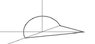# Stokes theorem, parametrizing composite curves

• Gauss M.D.

## Homework Statement

Calculate the line integral:

F = <xz, (xy2 + 2z), (xy + z)>

along the curve given by:

1) x = 0, y2 + z2 = 1, z > 0, y: -1 → 1
2) z = 0, x + y = 1, y: 1→0
3) z = 0, x-y = 1, y: 0 → -1

## The Attempt at a Solution

I don't think the problem is very difficult when just dividing the line integral into three parts, calculating each separately. But I want to be thorough to see if I got all the concepts.

I tried to draw the curve (see attachment) which made me realize a cone cut in half would be a capping surface, so we should be able to apply Stokes theorem. But I'm having trouble parametrizing it since we've basically dealt exclusively with very standard parametrizations.

I think that one parameter should be the height of the cone, h = $\sqrt{y^{2}+z^{2}$ running from 0 to 1 and the other should be the angle in the xz-plane running from 0 to $\pi$. I'm just having trouble setting up the variable substitution. Can anyone give me a push?

#### Attachments

•semicone.jpg
2.3 KB · Views: 309
Hi Gauss M.D.!Before you spend time trying to apply stokes …

what is the curl?<x-2,x-y,y^2>... you're saying Stokes theorem is a bad idea here?

stokes is useful if the curl is 0, or something simple

which do you think is easier, integrating that curl over that curved surface, or integrating the original line integral directly?